Summarize Within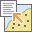The Summarize Within tool calculates statistics in areas where an input layer is within or overlaps a boundary layer. The area you are summarizing within can be an area layer or a hexagonal or square bin.

Workflow diagramAnalysis using GeoAnalytics Tools

Analysis using GeoAnalytics Tools is run using distributed processing across multiple ArcGIS GeoAnalytics Server machines and cores. GeoAnalytics Tools and standard feature analysis tools in ArcGIS Enterprise have different parameters and capabilities. To learn more about these differences, see Feature analysis tool differences.

Examples

• To complete routine maintenance projects efficiently, the city uses Summarize Within to count the street lights and to sum the miles of bike lanes within each maintenance assessment district. It can then estimate the material and staff needed to complete the work in each district.

• A cable provider is starting a pilot program that provides low-cost Internet access to low-income community college students. Summarize Within can be used to determine the number of low-income families in each college district so the cable provider can choose an appropriate district for its pilot program.

• A development company wants to create a new mixed-use project development in an urban center for the county. Within each city, the square area of potential development sites with good access to shops, restaurants, and light rail can be calculated using Summarize Within. This simplifies the site selection process.

Usage notes

The inputs for Summarize Within must be two layers. The first layer is the area used as a boundary to summarize your second layer; it's called the Summary Area and can be composed of an area layer you specify or square or hexagonal bins. The second layer specified is a point, line, or area layer to be summarized and is called the Summarized Layer.

Tip:

Depending on the configuration of your organization, you will have access to either Esri Living Atlas Analysis Layers, such as counties and hex bins, or Custom Analysis Layers. Click the drop-down arrow for the Choose area layer to summarize other features within its boundaries parameter to select an analysis layer to use as a boundary.

A Count of Points, Total Length, or Total Area box will appear depending on the type of features to summarize in your layer. The boxes are checked by default and can only be unchecked if statistics are being calculated. The default distance measure will depend on the units in your profile.

TotalInput featuresDefaultOptions

Count of Points

Points

None

None

Total Length

Lines

Miles (U.S. Standard setting) or Kilometers (Metric setting)

• Miles
• Feet
• Kilometers
• Meters
• Yards

Total Area

Areas

Square Miles (U.S. Standard setting) or Square Kilometers (Metric setting)

• Square Miles
• Square Kilometers
• Square Meters
• Hectares
• Acres

You can optionally calculate standard statistics. For lines and areas, all weighted statistics will be calculated. Both the standard summary field statistics and the weighted summary field statistics are applied to data for the features in the Summarized Layer that intersect the Summary Area layer. The weighted summary field statistics are multiplied by a weight based on the proportion of the Summary Area intersecting each feature in the Summarized Layer.

For standard statistics, there are eight options: count, sum, mean, minimum, maximum, range, standard deviation, and variance. There are two options for string statistics: count and any. There are six weighted statistics that are calculated on numeric fields in the layer to be summarized: count, sum, mean, minimum, maximum, and range.

Weighted statistics are not calculated for string data. Each time a Field and Statistic are specified, a row is added to the tool pane so more than one statistic can be calculated. You can view the summarized results in the result layer's table or pop-ups. By default, the count of features intersecting the Summary Area is always calculated.

Optionally, a Group By field can be selected so statistics are calculated separately for each unique attribute value. When a Group By field is selected, a summary table listing each feature and statistic by Group By field value will also be created.

The Add minority, majority and Add percentages check boxes are checked when a Group By field is selected. The minority and majority will be the least and most dominant value from the Group By field, respectively, where dominance is determined using the count of points, total length, or total area of each value.

When the Add minority, majority check box is checked, two fields will be added to the result layer. The fields will list the values from the Group By field that are the minority and majority for each result feature.

When the Add percentages check box is checked, two fields will be added to the result layer listing the percentage of the count of points, total length, or total area that belong to the minority and majority values for each feature. A percentage field will also be added to the result table listing the percentage of the count of points, total length, or total area that belong to all values from the Group By field for each feature.

It is important to consider the statistic you are calculating and what the data represents when choosing between standard and weighted statistics. For example, you may want to use weighted statistics for counts and amounts, and standard statistics for rates and indexes.

If Use current map extent is checked, only those features in the input layer and the layer to be summarized that are visible within the current map extent will be analyzed. If unchecked, all features in both the input layer and the layer to be summarized will be analyzed, even if they are outside the current map extent.

GeoAnalytics Tools analysis using binning (hexagon or square) with a specified geographic coordinate system will automatically use a projected coordinate system based on the extent of the data. To learn more about setting your coordinate system for analysis, see Use the analysis environments for GeoAnalytics Tools in Map Viewer.

Statistical calculations are computed using geodesic distances.

Limitation

• Only summary areas that intersect at least one feature in the layer that is summarized will be included in the results.

How Summarize Within works

Equations

For summarized line and area features, weighted statistics incorporate Summary Area weights. None of the statistics for point features are weighted. The following table shows the equations used to calculate variance and the weighted mean.

StatisticEquationVariablesFeatures

Variance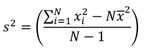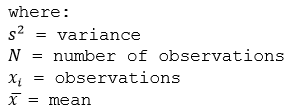Points

Weighted Mean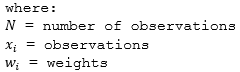Weights are calculated as the percentage of the feature i within the summary area.

Lines and Areas

Points

Point layers are summarized using only the point features that fall within the Summary Area. Weighted statistics cannot be applied when summarizing points.

The figure and table below explain the statistical calculations of a point Summarized Layer within hypothetical areas. The Population field was used to calculate the statistics (Count, Sum, Minimum, Maximum, Range, Mean, Standard Deviation, and Variance) for the layer.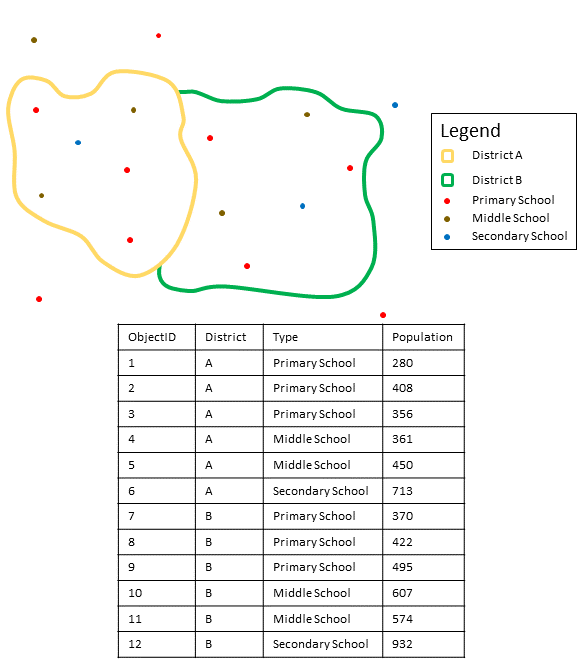Point layers are summarized using only points located within the area layer. An example attribute table is shown above with values to be used in hypothetical statistic calculations.
Numeric statisticResults District A

Count

Count of:

[280, 408, 356, 361, 450, 713] = 6

Sum

280 + 408 + 356 + 361 + 450 + 713 = 2,568

Minimum

Minimum of:

[280, 408, 356, 361, 450, 713] = 280

Maximum

Maximum of:

[280, 408, 356, 361, 450, 713] = 713

Range

713 - 280 = 433

Mean

2568/6 = 428

Variance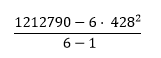= 22737.2

Standard Deviation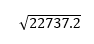= 150.7886
String statisticResults District A

Count

= 6

Any

= Secondary School

Note:

The count statistic (for strings and numeric fields) counts the number of nonnull values. The counts of [0, 1, 10, 5, null, 6] = 5. The count of [Primary, Primary, Secondary, null] = 3.

A real-life scenario in which this analysis could be used is in determining the total number of students in each school district. Each point represents a school. The Type field gives the type of school (elementary, middle school, or secondary) and a student population field gives the number of students enrolled at each school. The calculations and results are given for District A in the table above. From the results, you can see that District A has 2,568 students. When running the Summarize Within tool, the results would also be given for District B.

Lines

For weighted statistics, line layers are summarized using only the proportions of line features that are within the Summary Area. Standard (non-weighted) statistics summarize any line intersecting the Summary Area. When summarizing lines using weighted statistics, use counts and amounts (rather than rates or indices) so proportional calculations make logical sense in your analysis.

The figure and table below explain the statistical calculations of a line Summarized Layer within a hypothetical Summary Area. The Volume field was used to calculate the statistics (Count, Sum, Minimum, Maximum, Range, Mean, Standard Deviation, and Variance) for the layer. The standard statistics are calculated using lines that intersect the boundary and the weighted statistics are calculated using the proportion of the lines that are within the Summary Area. Standard Deviation and Variance are only available for standard statistics.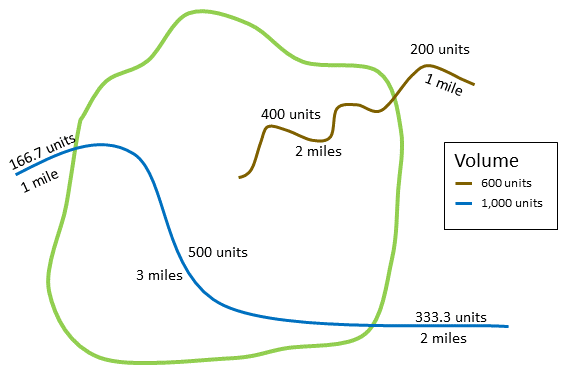Line layers are summarized using standard statistics and weighted statistics as shown below
Numeric statisticsStandard statisticsWeighted statistics

Calculating Weights

Not applicable

Weight of the brown line (value = 600):

2/3 = .3333

Weight of the blue line (value = 1000):

3/6 = .5

Count

Count of:

[1000, 600] = 2

Count of:

1 x (3/6) + 1 x (2/3) = 1.667

Sum

1000 + 600 = 1600
1000 x (3/6) + 600 x (2/3) = 900

Minimum

Minimum of:

[1000, 600] = 600

Minimum of:

[1000 x (3/6), 600 x (2/3)]
[500, 400] = 400

Maximum

Maximum of:

[1000, 600] = 1000

Maximum of:

[1000 x (3/6), 600 x (2/3)]
[500, 400] = 500

Range

1600 - 600 = 1000
500 - 400 = 100

Mean

(1000 + 600)/2 = 800
(1000 x (3/6) + 600 x (2/3))/(3/6 + 2/3)
(500 + 400)/(4/6) = 1350

Variance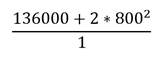= 80000

Not applicable

Standard Deviation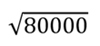= 282.8427

Not applicable

A real-life scenario in which this analysis could be used is in determining the total volume of water in rivers within the boundaries of a state park. Each line represents a river that is partially located inside the park. From the results, you can see that there are 5 miles of rivers within the park and the total volume is 900 units.

Areas

Area layers are summarized using only the proportions of the area features that are within the input boundary. When summarizing areas, use fields with absolute numbers so proportional calculations make logical sense in your analysis. The results layer will be displayed using graduated colors.

Weighted statistics for summarized area layers are based on the proportions of the Summary Area features that are within the Summarized Layer. When summarizing areas, use counts or amounts (rather than rates or indices) so proportional calculations make logical sense in your analysis.

The figure and table below explain the statistical calculations of an area layer within a hypothetical Summary Area. The population field was used to calculate the statistics (Count, Sum, Minimum, Maximum, Range, Mean, Standard Deviation, and Variance) for the layer. The standard statistics are calculated using areas that intersect the Summary Area, and the weighted statistics are calculated using a proportional weight based on the portion of summary areas contained within each Summarized Layer. Standard Deviation and Variance are only available for standard statistics.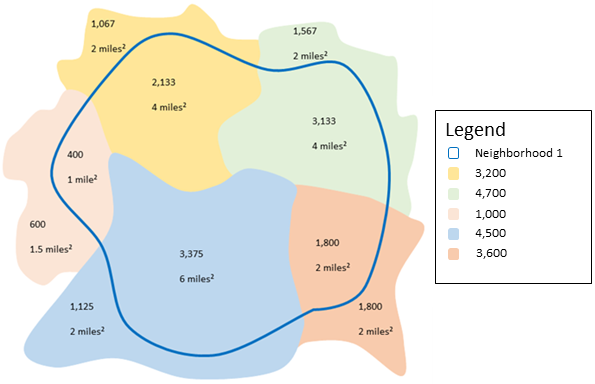Summary statistics are computed for areas in the Summarized Layer that intersect the summary areas. Weights for weighted statistics are based on the proportion of the summary areas that overlap the summarized layer features.
Numeric statisticsStandard statistics: Results Neighborhood 1Weighted statistics: Results Neighborhood 1

Calculating Weights

Weight of the yellow area (value = 3200):

4/(2+4) = 3/4

Weight of the green area (value = 4700):

4/(2+4) = 2/3

Weight of the pink area (value = 1000):

1/(1+1.5) = 2/5

Weight of the blue area (value = 4500):

6/(2+6) = 3/4

Weight of the orange area (value = 3600):

2/(2+2) = 1/2

Count

Count of:

[3200, 4700, 1000, 4500, 3600] = 5

Count of:

(3/4)+(2/3)+ (2/5)+(3/4)+ (1/2) = 3.06667

Sum

3200 + 4700 + 1000 + 4500 + 3600 = 17000
(3/4) x 3200 +(2/3) x 4700 + (2/5) x 1000 +(3/4) x 4500 + (1/2) x 3600 = 11108.33

Minimum

Minimum of:

[3200, 4700, 1000, 4500, 3600] = 1000

Minimum of:

[(3/4) x 3200, (2/3) x 4700, (2/5) x 1000, (3/4) x 4500, (1/2) x 3600]
[2400, 3133.33, 400, 3375, 1800] = 400

Maximum

Maximum of:

3200, 4700, 1000, 4500, 3600] = 4700

Maximum of:

[2400, 3133.33, 400, 3375, 1800] = 3375

Range

4700 - 1000 = 3700
3,375 - 400 = 2,975

Mean

(17000)/5 = 3400
(11108.33)/[3.06667] = 3622.83

Variance= 2185000

Not applicable

Standard Deviation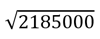= 1478.175

Not applicable

ArcGIS API for Python example

The Summarize Within tool is available through ArcGIS API for Python.

This example calculates the distance and average slope of bike lanes within each city district.

# Import the required ArcGIS API for Python modules
import arcgis
from arcgis.gis import GIS
from arcgis.geoanalytics import summarize_data

# Connect to your ArcGIS Enterprise portal and check that GeoAnalytics is supported
portal = GIS("https://myportal.domain.com/portal", "gis_publisher", "my_password", verify_cert=False)
if not portal.geoanalytics.is_supported():
print("Quitting, GeoAnalytics is not supported")
exit(1)

# Find the big data file share dataset you're interested in using for analysis
search_result = portal.content.search("", "Big Data File Share")

# Look through search results for a big data file share with the matching name
bd_file = next(x for x in search_result if x.title == "bigDataFileShares_CityData")

# Look through the big data file share for BikeLanes
bike_lanes = next(x for x in bd_file.layers if x.properties.name == "BikeLanes")

# Look through the big data file share for districts
districts = next(x for x in bd_file.layers if x.properties.name == "districts")

weighted_summary_fields = [{"statisticType" : "Average","onStatisticField" : "Slope"}]

# Run the tool Summarize Within
summarize_within_result = summarize_data.summarize_within(summary_polygons = districts,
summarized_layer = bike_lanes,
weighted_summary_fields = weighted_summary_fields,
output_name = "summary_of_bike_lanes")

# Visualize the tool results if you are running Python in a Jupyter Notebook
processed_map = portal.map('Your City, State', 10)
processed_map

Similar tools

Use Summarize Within to calculate statistics for features that overlap a boundary layer. Other tools may be useful in solving similar but slightly different problems.

Map Viewer analysis tools

If you are trying to summarize points and want to apply time stepping, use the Aggregate Points tool.

ArcGIS Desktop analysis tools

This GeoAnalytics Tools is available in ArcGIS Pro.

Summarize Within performs the functions of the Spatial Join and Summary Statistics tools.